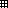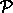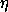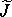# Index

 Any inaccuracies in this index may be explained by the fact that it has been prepared with the help of a computer. Donald E. Knuth, Fundamental Algorithms (Volume 1 of The Art of Computer Programming)

Each index entry links to the first occurrence of that entry in the book. Bracketed numbers (starting from 2) following the index entry link to the second and subsequent occurrences.

Baker, Henry, see Baker-Campbell-Hausdorff formula
Baker-Campbell-Hausdorff formula
Banana, see Book
Barrow-Green, June
Basin of attraction
Bicycle wheel
Birkhoff, George David, see Poincaré-Birkhoff theorem
bisect (bisection search), 
Body components of vector
Boltzmann, Ludwig, , 
Book
banana-like behavior of
rotation of, 
for down tuples
for functional arguments
Bulirsch-Stoer integration method
bulirsch-stoer
Butterfly effect

C (canonical phase-space transformation)
C (local-tuple transformation)
Campbell, John, see Baker-Campbell-Hausdorff formula
Canonical equations, see Hamilton's equations
Canonical heliocentric coordinates
Canonical perturbation theory, see Perturbation theory
Canonical plane
classical gauge
composition of, , 
conditions for
for driven pendulum
general
group properties of
for harmonic oscillator
invariance of antisymmetric bilinear form under
invariance of phase volume under
invariance of Poisson brackets under
as Lie series
Lie transforms, see Lie transforms
point transformations, see Point transformations
polar-canonical, see Polar-canonical transformation
to rotating coordinates, 
symplectic-matrix test
time evolution as
time-dependent
time-independent
canonical?
Cantori, 
car
Cartan, Élie, see Poincaré-Cartan integral invariant
Cauchy, Augustin Louis
cdr
Celestial objects, see also Asteroids; Comets; Earth; Galaxy; Hyperion; Jupiter; Mercury; Moon; Phobos; Planets
rotation of, , 
Center of mass
in two-body problem
Jacobi coordinates and
kinetic energy and
vector angular momentum and
Central force
collapsing orbits
epicyclic motion
gravitational
in 2 dimensions, , 
in 3 dimensions
Lie series for motion in
reduced phase space for motion in
Central potential, see Central force
Chain rule
for derivatives, 
for partial derivatives, 
for total time derivatives
for variations
homoclinic tangle and
in Hénon-Heiles problem
in spin-orbit coupling, 
near separatrices, , 
of Hyperion, 
of non-axisymmetric top
of periodically driven pendulum, 
overlapping resonances and
Characteristic exponent
Characteristic multiplier
Chart function ()
Chirikov, Boris V.
Chirikov-Taylor map
Church, Alonzo
Classical gauge transformations
Colon, names starting with
Comets, rotation of
Comma in tuple
islands and
of pendulum period with drive, 
periodic orbits and, 
rational rotation number and
small denominators and
of some tuple multiplication
of variation () with differentiation and integration
Commutator
of angular-momentum Lie operators
Jacobi identity for
of Lie derivative
Poisson brackets and
Compatible shape
compatible-shape
component, 
compose
Composition
of canonical transformations, , 
of functions, , 
of Lie transforms
of linear transformations
of operators
of rotations
Compositional canonical
compositional-canonical?
Compound data in Scheme
cond
Conditionals in Scheme
Configuration
Configuration manifold
Configuration path, see Path
Configuration space
Configuration-path function (), 
Conjugate momentum
non-uniqueness of
cons
Consequent in conditional
angular momentum, , , 
coordinate choice and
cyclic coordinates and
energy, , 
Jacobi constant
Lyapunov exponents and
momentum
Noether's theorem
phase space reduction and
phase volume, see Phase-volume conservation
Poisson brackets of
symmetry and, 
for top
Constant of motion (integral of motion), see also Conserved quantities; Hénon-Heiles problem
Constraint force
Constraint(s)
augmented Lagrangian and, 
configuration space and
as coordinate transformations
explicit
in extended bodies
holonomic, 
integrable, 
linear in velocities
nonholonomic (non-integrable)
on coordinates
rigid
as subsystem couplers
total time derivative and
velocity-dependent
velocity-independent
Constructors in Scheme
Contact transformation, see Canonical transformations
Continuation procedure
Continued-fraction approximation of irrational number
Contraction of tuples
coordinate
Coordinate function ()
Coordinate singularity
Coordinate transformations
constraints as
action-angle, see Action-angle coordinates
conserved quantities and choice of
constraints on
cyclic, 
heliocentric
ignorable (cyclic)
Jacobi
polar, see Polar coordinates
redundant, and initial conditions
rotating, see Rotating coordinates
spherical
Coordinate-independence
of action, 
of Lagrange equations, 
of variational formulation, 
Correction fluid
Cotangent space, bundle
Coupling systems
Coupling, spin-orbit, see Spin-orbit coupling
Curves, invariant, see Invariant curves
Cyclic coordinate, 

D, see Derivativevs. D
D (Scheme procedure for derivative),, see Partial derivative
Dt (total time derivative)
d'Alembert-Lagrange principle (Jean leRond d'Alembert)
Damped harmonic oscillator
define
Definite integral
definite-integral
Definitions in Scheme
Degrees of freedom
Delta function
as operator
as Poisson bracket
chain rule, 
in Scheme programs: D, 
notation: D, 
of function of multiple arguments, 
of function with structured arguments
of function with structured inputs and outputs
of state
partial, see Partial derivative
precedence of, 
with respect to a tuple
determinant
Differentiable manifold
Dimension of configuration space
Dissipation of energy
in free-body rotation
tidal friction
Dissipative system, phase-volume conservation
Dissolution of invariant curves, 
Distribution functions
Divided phase space, , 
Division in Scmutils
by a structure
generic character of
of vector by matrix
Dot notation
down, 
Down tuples
Driven harmonic oscillator
Driven pendulum, see Pendulum (driven)
Driven rotor, 
Dt (total time derivative)
Dynamical state, see State

F1(t, q, q')
F2(t, q, p')
F3(t, p, q')
F4(t, p, p')
F->C, 
F->CT
Fermat, Pierre, 
Fermat's principle (optics), 
Fermi, Enrico
Feynman, Richard P.
find-path
First amendment, see Degrees of freedom
First integral
elliptic, 
equilibria or periodic motion and, 
for Hamiltonian systems
hyperbolic, 
linear stability of
manifolds for
parabolic
Poincaré-Birkhoff fixed points
Poincaré-Birkhoff theorem
rational rotation number and
Floating-point numbers in Scheme
Floquet multiplier
Flow, defined by vector field
Force
central, see Central force
exerted by constraint
Forced libration of the Moon
Forced rigid body, see Rigid body, forced
Formal parameters
of a function
of a procedure
frame
Free libration of the Moon
Free particle
action
Lagrange equations for
Lagrangian for
Free rigid body, see Rigid body (free)
Freudenthal, Hans
Friction
internal
tidal
Function definition
Function(s)
arithmetic operations on, 
composition of, , 
homogeneous
operators vs., 
orthogonal, tuple-valued
parallel, tuple-valued
selector, 
tuple of, 
vs. value when applied, 
with multiple arguments, , 
with structured arguments, , 
with structured output, 
Functional arguments
Functional mathematical notation, 
Fundamental Poisson brackets

Ii, Iz (selector), 
if
Ignorable coordinate, see Cyclic coordinate
Indexing, zero-based, see Zero-based indexing
Inertia tensor
diagonalization of
kinetic energy in terms of
principal axes of
transformation of
Inertia, moments of, see Moment(s) of inertia
Initial conditions, see Sensitivity to initial conditions; State
Inner product of tuples
free-body rotation
Integers in Scheme
Integrable constraints, 
Integrable systems
periodic orbits of near-integrable systems
perturbation of, , 
surfaces of section for
Integral invariants
generating functions and
Poincaré
Poincaré-Cartan, 
Integral, definite
Integration, see Numerical integration
Invariant curves, 
dissolution of, 
finding (computing)
finding (strategy)
irrational rotation number and
Kolmogorov-Arnold-Moser theorem
Irrational number, continued-fraction approximation
for Hénon-Heiles problem
for periodically driven pendulum, , 
for standard map
perturbative vs. actual
in Poincaré-Birkhoff construction
Poisson series and
secondary, 
size of, 
small denominators and, 
Iteration in Scheme

KAM theorem, see Kolmogorov-Arnold-Moser theorem
Kepler problem
in reduced phase space
solution via Hamilton-Jacobi equation
Kepler's third law
Kepler, Johannes, see Kepler...
Kinematics of rotation
Kinetic energy
ellipsoid of
in Lagrangian
of axisymmetric top
of free rigid body
rotational and translational
in spherical coordinates
Knuth, Donald E.
Kolmogorov, A. N., see Kolmogorov-Arnold-Moser theorem
Kolmogorov-Arnold-Moser theorem, 

M->omega-body
M-of-q->omega-of-t
MacCullagh's formula
make-path, 
Manifold
differentiable
stable and unstable
Map
area-preserving
Chirikov-Taylor
Poincaré
representation in programs
standard
symplectic
twist
Mars, see Phobos
Mass point, see Point mass
Mathematical notation, see Notation
Mather, John N. (discoverer of sets named cantori by Ian Percival)
Matrix
orthogonal
Pauli spin
symplectic, , 
as tuple
Maupertuis, Pierre-Louis Moreau de, 
Mean motion
Mechanics
Newtonian vs. variational formulation, 
Mercury, resonant rotation of, 
Minimization
of action
in Scmutils, 
minimize
Moment(s) of inertia
principal
of top
conjugate to coordinate, see Conjugate momentum
conservation of
generalized, see Generalized momentum
variation of
momentum
Momentum path
Momentum state function ()
Moon
history of
libration of, , 
rotation of, , , 
Moser, Jürgen, see Kolmogorov-Arnold-Moser theorem
Motion
atomic-scale
chaotic, see Chaotic motion
dense, on torus
deterministic
epicyclic
ergodic
periodic, see Periodic motion
quasiperiodic, see Quasiperiodic motion
realizable vs. conceivable
smoothness of
tumbling, see Chaotic motion, of Hyperion; Rotation(s), (in)stability of
multidimensional-minimize, 
Multiplication of operators as composition
Multiplication of tuples
as composition
as contraction
Multiply-periodic functions, Poisson series for
Multipole expansion of potential energy

Oblateness
*ode-integration-method*
omega (symplectic 2-form)
omega-cross
Operator
arithmetic operations on, 
exponential identities
function vs., 
generic
Operators
derivative (D), see Derivative
Euler-Lagrange ( E )
Lie derivative (LH)
Lie transform (E', W)
partial derivative (), see Partial derivative
variation ()
Optical libration of the Moon
Optics
Fermat
Snell's law
Orbit, see Phase-space trajectory
Orbital elements
Lagrange equations for
retrodiction of
Euler's equations and
nonsingular coordinates for
specified by Euler angles
specified by rotations
Orientation vector
Orthogonal matrix
Orthogonal transformation, see Orthogonal matrix
Orthogonal tuple-valued functions
Oscillator, see Harmonic oscillator
osculating-path
Osculation of paths
Out-of-roundness parameter

S or S(action)
vs.(action)
s->m (structure to matrix)
s->r (spherical-to-rectangular)
Salam, Abdus
Saturn, see Hyperion
for Gnu/Linux, where to get it
Schrödinger, Erwin, 
division by a structure
division of vector by matrix
generic arithmetic, , 
minimization, 
numerical integration, , 
operations on operators
simplification of expressions, 
where to get it
Second law of thermodynamics
Section, surface of, see Surface of section
Secular terms in perturbation theory
elimination of
Selector function, 
Selectors in Scheme
Semicolon in tuple, 
chaos near, , 
motion near
series
series:for-each
show-expression
Shuffle functionSimplification of expressions, 
Singularities, , 
nonsingular generalized coordinates
Sleeping top
Small denominators
for periodically driven pendulum
in perturbation theory, 
resonance islands and, 
Small divisors, see Small denominators
Snell's law
Solvable systems, see Integrable systems
Spherical coordinates
kinetic energy in
Lagrangian in
Spin matrices, Pauli
Spin-orbit coupling
chaotic motion, 
Lagrange equations for
Lagrangian for
periodically driven pendulum and
perturbation theory for
surface of section for
Spivak, Michael, 
square
for tuples, 
Stability, see Equilibria; Instability; Linear stability
Stable manifold
computing
Standard map
standard-map
Stars, see Galaxy
State
evolution of, see Time evolution of state
Hamiltonian vs. Lagrangian
in terms of coordinates and momenta (Hamiltonian)
in terms of coordinates and velocities (Lagrangian)
State derivative
Hamiltonian
Hamiltonian vs. Lagrangian
Lagrangian
State path
Hamiltonian
Lagrangian
State tuple
Stationarity condition
Stationary action, see Principle of stationary action
Stationary point
Steiner's theorem
computing
Subscripts
down and
for down-tuple components
for momentum components, 
for selectors
Summation convention
Superscripts
for coordinate components, , 
for up-tuple components
for velocity components, 
up and
Surface of section
in action-angle coordinates
area preservation of, 
computing (Hénon-Heiles)
computing (stroboscopic)
fixed points, see Fixed points
for autonomous systems
for Hénon-Heiles problem
for integrable system
for non-axisymmetric top
for periodically driven pendulum, , , 
for spin-orbit coupling
invariant curves, see Invariant curves
islands, see Islands in surfaces of section
stroboscopic
Symbolic values
Symbols in Scheme
Symmetry
conserved quantities and, 
continuous
of Lagrangian
of top
Symplectic bilinear form (2-form)
invariance under canonical transformations
Symplectic condition
Symplectic integration
Symplectic map
Symplectic matrix, , 
antisymmetric bilinear form and
Poisson brackets and
Symplectic unit J, Jn, 
symplectic-matrix?
symplectic-transform?
symplectic-unit
symplectic?
Syntactic sugar
System derivative, see State derivative[]
T-rigid-body
Taylor, J. B.
Tensor arithmetic
notation and, 
summation convention
tuple arithmetic vs., 
Theology and principle of least action
Thermodynamics, second law
Three-body problem, restricted
Tidal friction
time
Time evolution of state
action and, 
as canonical transformation
in phase space
Poincaré-Cartan integral invariant and
time-independent-canonical?
Time-dependent canonical transformations
energy conservation and
Time-independent canonical transformations
Top
Axisymmetric, see Axisymmetric top
non-axisymmetric
Top banana, see Non-axisymmetric top
Torque
in Euler's equations
in spin-orbit coupling
Total time derivative
affecting conjugate momentum
commutativity of
computing
constraints and
identifying
notation: Dt
Trajectory, see Path; Phase-space trajectory
Transformation
canonical, see Canonical transformations
coordinate, see Coordinate transformations
Legendre, see Legendre transformation
Lie, see Lie transforms
orthogonal, see Orthogonal matrix
point, see Point transformations
symplectic, see Symplectic transformations
True anomaly
Tumbling, see Chaotic motion, of Hyperion; Rotation(s), (in)stability of
Tuples
arithmetic on, 
commas and semicolons in
component selector: Ii, Iz, 
composition and
contraction
of coordinates
down and up
of functions, 
inner product
linear transformations as
local, see Local tuple
matrices as
multiplication of
rotations as
squaring, 
state tuple
up and down
Twist map
Two-body problem
Two-trajectory method

Vakonomic mechanics
Variation
chain rule
of a function
of a path, , 
of action
operator:Variational equations
Variational formulation of mechanics, 
Variational principle, see Principle of stationary action
Vector
body components of
in Scheme
square of
vector